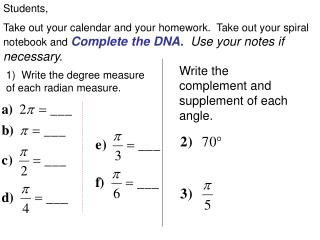# Students, - PowerPoint PPT PresentationDownload PresentationStudents,

Students,
Download Presentation## Students,

- - - - - - - - - - - - - - - - - - - - - - - - - - - E N D - - - - - - - - - - - - - - - - - - - - - - - - - - -
##### Presentation Transcript

1. Students, Take out your calendar and your homework. Take out your spiral notebook and Complete the DNA.Use your notes if necessary. Write the complement and supplement of each angle. 1) Write the degree measure of each radian measure.

2. Definition of Trigonometric Functions Let t be a real number and let (x, y) be the point on the unit circle corresponding to t.

3. Determine the exact values of the six trigonometric functions of the angle θ.

4. Find the indicated trig ratio below.

5. Use a calculator to find the trig ratios.

6. 1) A circle has a radius of 12 inches. Find the length of the arc intercepted by a central angle of 125°. 2) A circle has a diameter of 8 inches. Find the length of the arc intercepted by a central angle of 270°.

7. Linear and Angular Speed • 2) The circular blade on a saw rotates at 1200 revolutions per minute. • Find the angular speed in radians per second. • The blade has a radius of 6 inches. Find the linear speed of a blade tip in inches per second.

8. Linear and Angular Speed • 2) The circular blade on a saw rotates at 2400 revolutions per minute. • Find the angular speed in radians per second. • The blade has a radius of 4 inches. Find the linear speed of a blade tip in inches per second.